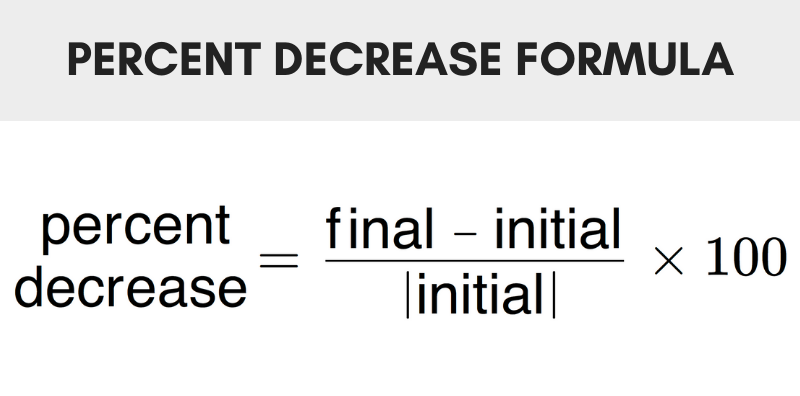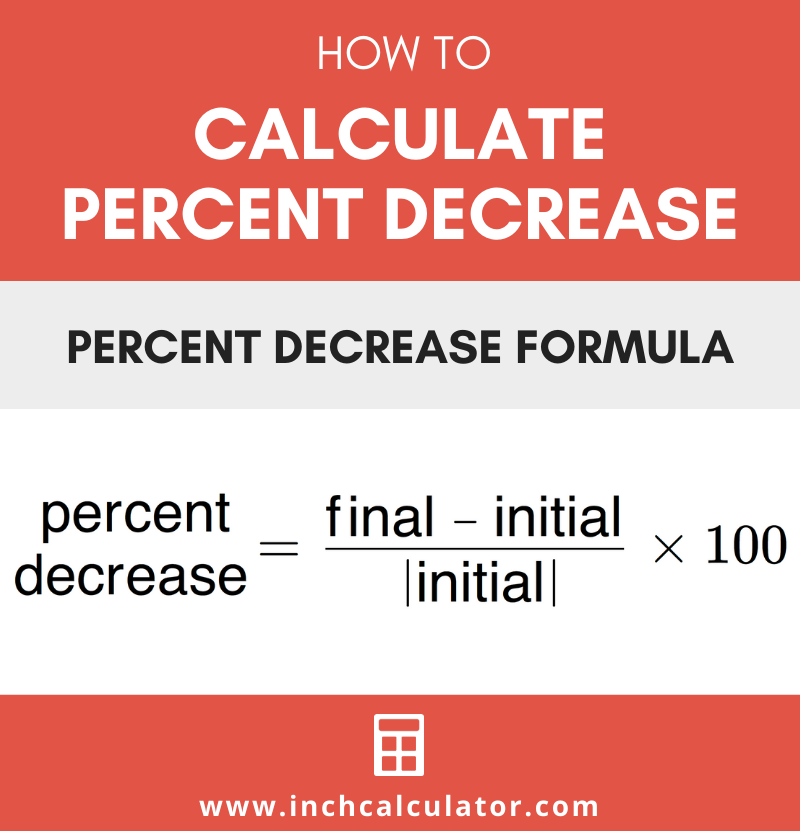# Percent Decrease Calculator – Find Percentage Decrease

Find the percent decrease or the decrease in a value by entering the known values below.

%

## Result:

60% decrease

### Steps to Solve Percent Decrease

p=(y2 - y1)|y1|×100
Substitute values in the equation
p=
(120 - 300)|300|×100
Simplify
p=
-180300×100
p=-0.6×100
p=-60%

## How to Find Percent Decrease

Percent decrease is the reduction of a value expressed as a percentage. It is the percentage of change from an initial to a smaller final value.

The percentage of decrease is found using a formula.

### Percent Decrease Formula

The formula to calculate the percentage of decrease is the difference between the initial and final value divided by the initial value.

p = final – initial|initial| × 100

Thus, the decrease in value is equal to the final value minus the initial value, divided by the absolute value of the initial. Multiply by 100 to find the percentage.For example, let’s calculate the percentage of reduction from 120 to 80.

p = 80 – 120|120| × 100
p = -40120 × 100
p = -0.3333 × 100
p = -33.33%

## How to Calculate Reduction by a Percentage

When the percent decrease is known, you can calculate the reduction of a value by multiplying the initial value by the percentage and adding it to the initial value.

final = initial – (initial × p100)

The final value is equal to the initial value times the reduction percentage divided by 100, plus the initial value.

For example, let’s calculate the reduction of 150 by 20%

final = 150 – (150 × 20100)
final = 150 – (150 × .2)
final = 150 – 30
final = 120

Use our percent increase calculator to find the increase in a value.